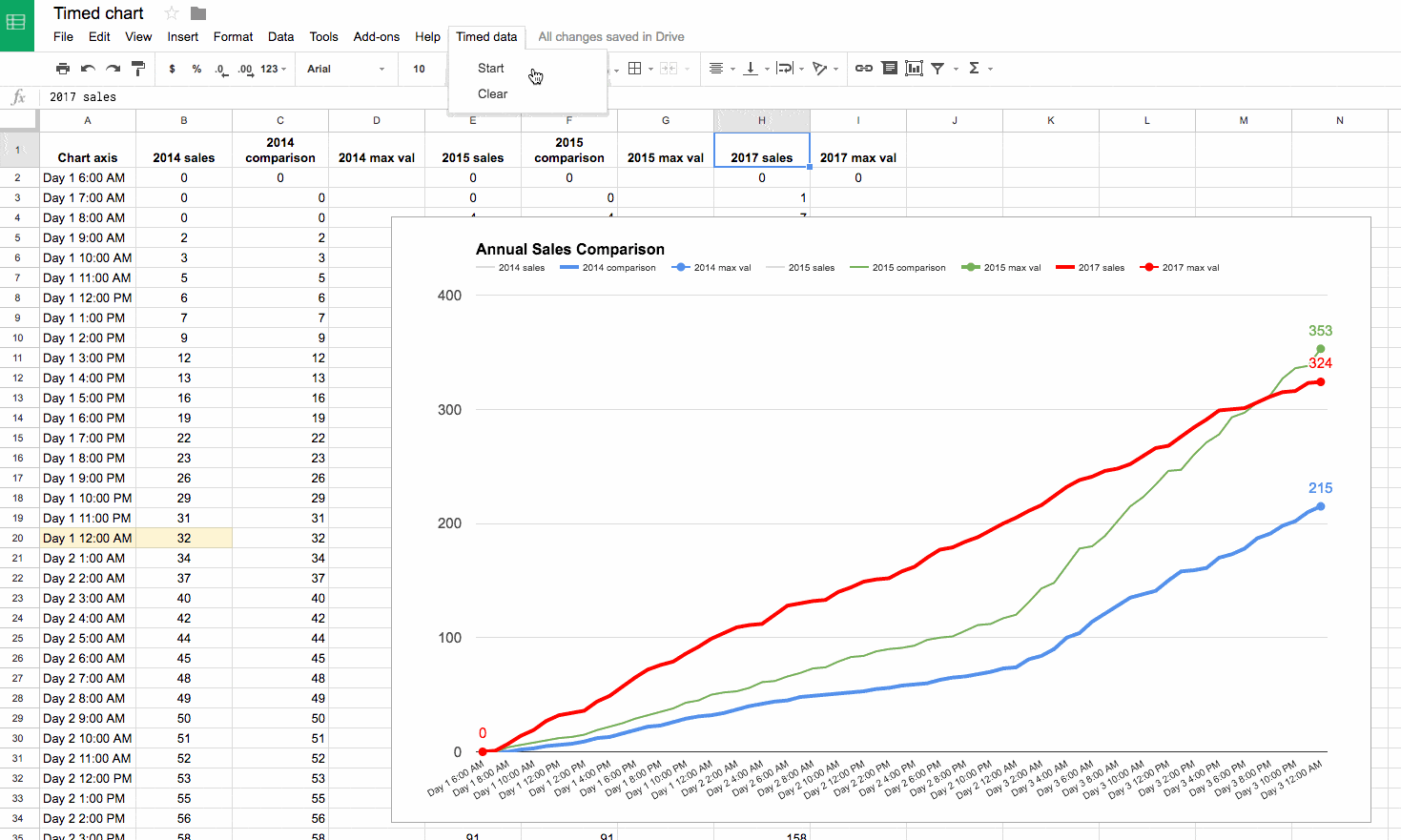## Multi-colored line charts in Google Sheets

In this article, you’ll see how to create a multi-colored line chart in Google Sheets, for example when the line is increasing it’s colored green, when it’s decreasing it’s colored red, as shown in this image: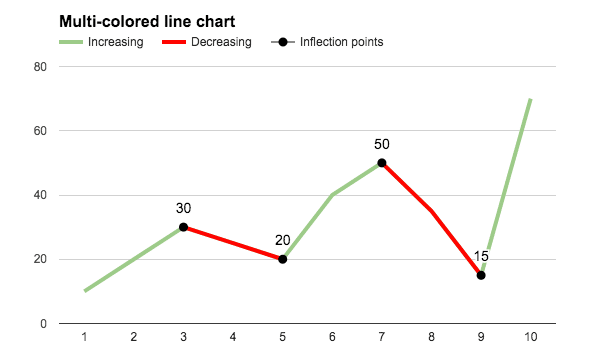The embedded charts tool in Google Sheets is pretty basic, so we can only achieve this with a formula workaround.

## How do I create a multi-colored line chart in Google Sheets?

### Basic Example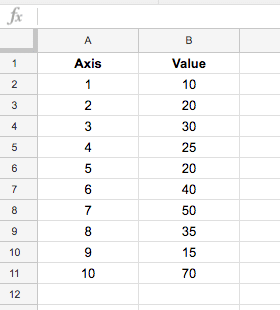which, when charted, looks like this: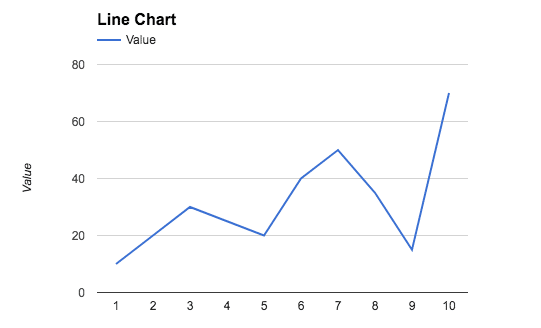To create the colored version seen at the top of this post, we need to add helper columns to the dataset, one to create a dataset of decreasing values, and an optional column to mark the inflection points (where the line changes from going up to going down, or vice versa).

The finished dataset looks like this: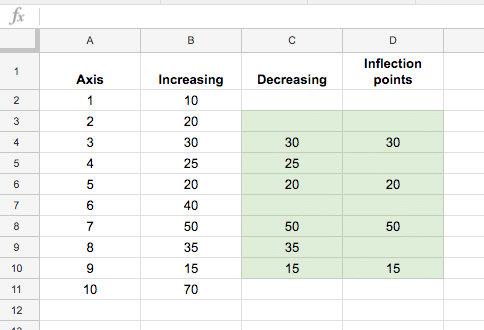The green highlighted cells contain formulas to calculate the decreasing data and the inflection points (see below). The first and last lines in column C and D (cells C2, D2, C11, D11 in this case) are left blank.

The formula in column C, starting in cell C3 down to C10 is:

`=IF(OR(B3>B4,B3<B2),B3,"")`

The formula in column D, for identifying inflection points, starting in cell D3 down to D10, is:

`=IF(OR(B3=MAX(B2:B4),B3=MIN(B2:B4)),B3,"")`

With this data table setup, highlight the whole table (use Ctrl + A, or Cmd + A on a Mac, to do this quickly) and `Insert > Chart...`: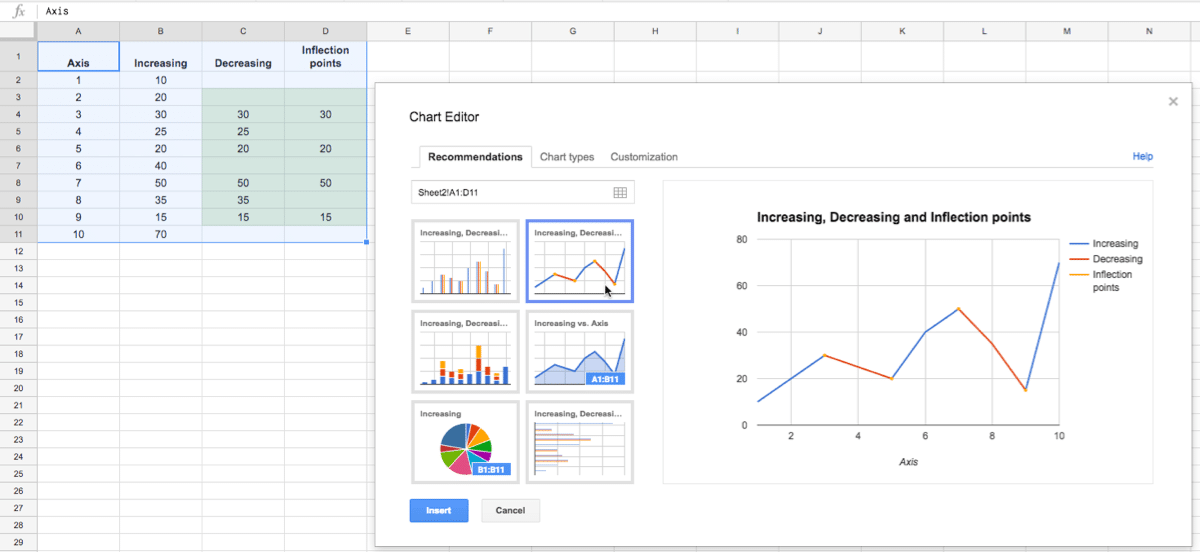Then simply format to the style you want, such as coloring the Increasing Series in green and the Decreasing Series in red:### Problem with this basic interpretation of this chart

This method has a drawback though, if you have adjacent inflection points, i.e decreasing – increasing – decreasing, then it tricks the chart so it colors the whole section decreasing, as shown in this image: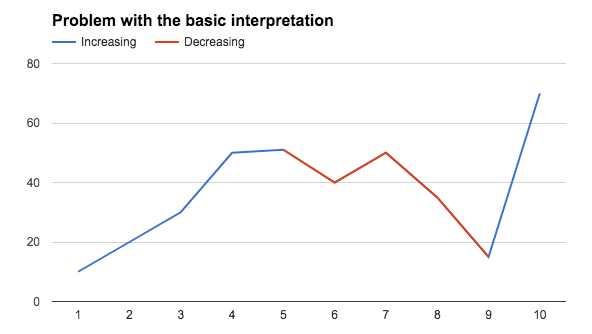### The fix

If you encounter this issue of adjacent inflection points, then you’ll need to create additional decreasing series to separate them, like this example dataset: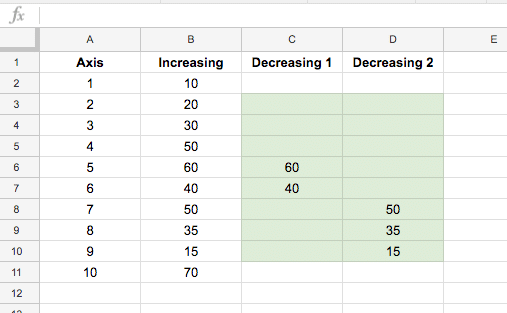The final chart will then look like this: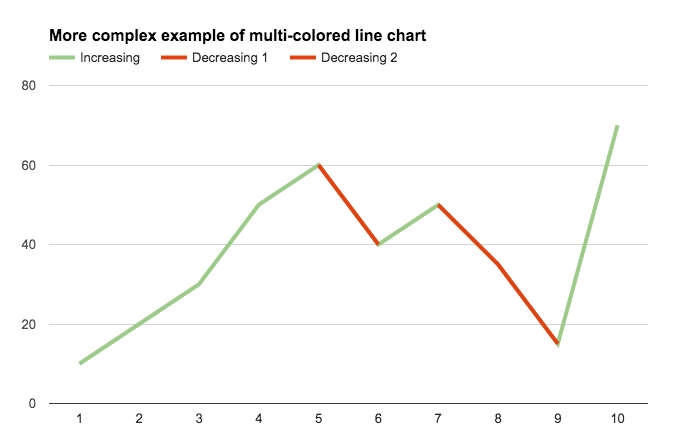Again we need to split the inflection point data into two columns so there are no adjacent inflection points in these series. The dataset now looks like: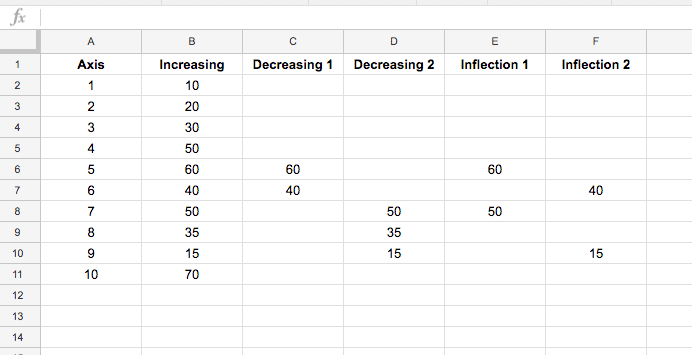and the final chart: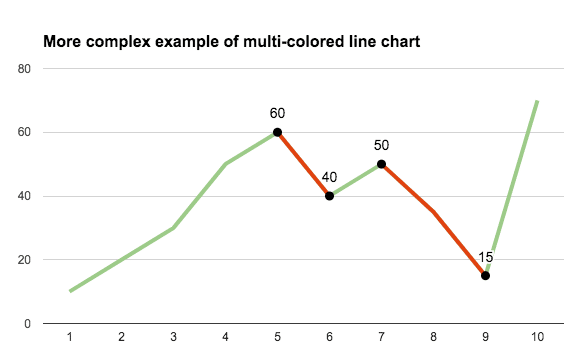### Even more customization options

Select the Combo chart instead of the straightforward line chart and change the increasing series to a line and the decreasing series to area charts: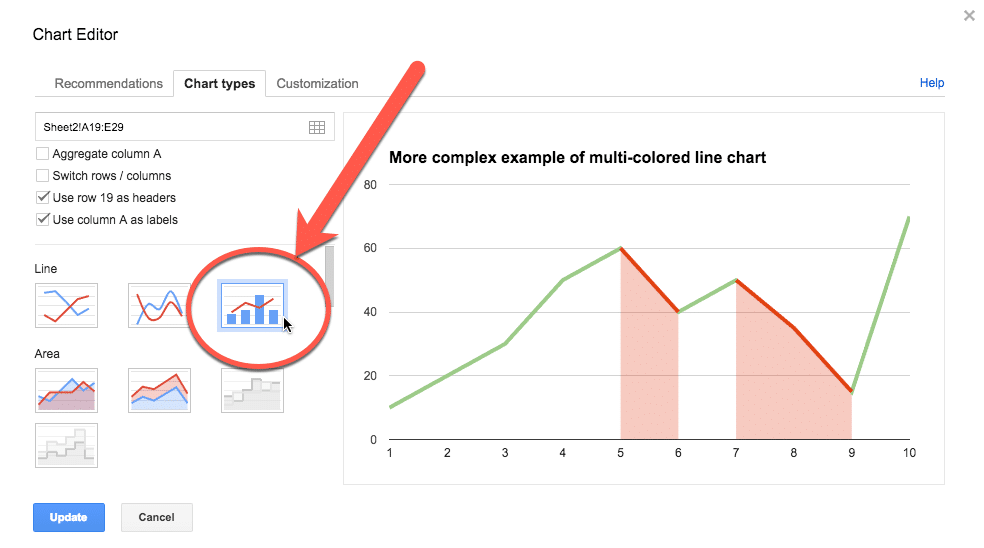Your final chart will look like this: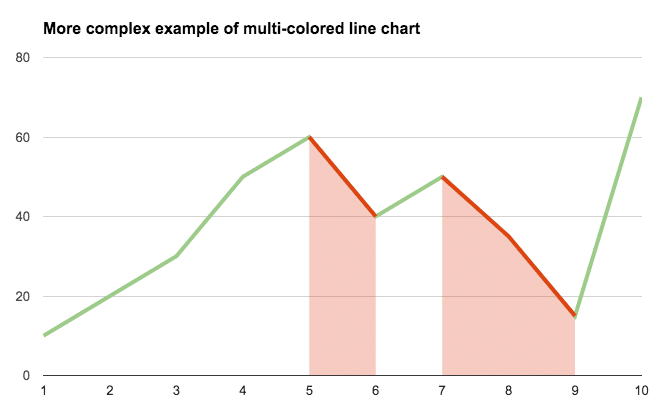And here’s the version with the inflection points marked: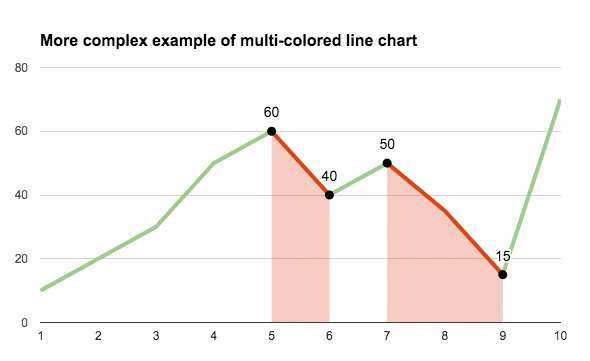## Can I see an example worksheet?

Yes, here you go.

## Reverse text in a cell in Google Sheets

In this example, I enter some text into cell A1 and a formula in cell B1 reverses the order of the text: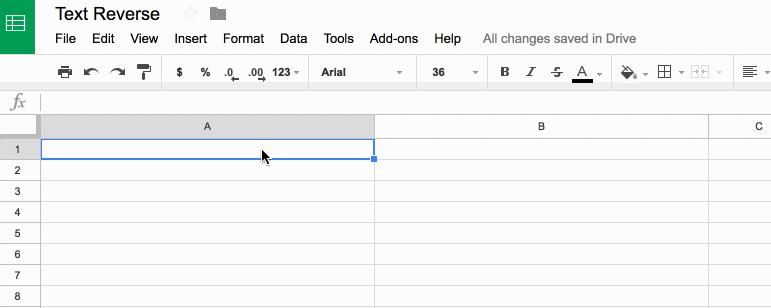# How to reverse the order of a text string

## What’s the formula?

`=ArrayFormula(IFERROR(PROPER(CONCATENATE(MID(A1,LEN(A1)-ROW(INDIRECT("1:"&LEN(A1)))+1,1))),""))`

It’s a beast! See below for a detailed breakdown of how it works.

It might be useful if you wanted to find the last character in a string, or the last occurrence of a character using the FIND() function.

Here’s an alternative, which reverses the capitalization too (submitted by Michael D over email – thanks!):

`=JOIN("",ARRAYFORMULA(MID(A1,LEN(A1)-ROW(INDIRECT("1:"&LEN(A1)))+1,1)))`

## How does this formula work?

Basically we make an array of numbers corresponding to how many letters are in the original text string. Then we reverse that, so the array shows the largest number first. Then we extract each letter at that position (so the largest number will extract the last letter, the second largest will extract the second-to-last letter, etc., all the way to the smallest number extracting the first letter). Then we concatenate these individual letters.

Easy! Err…

The only way to really understand this formula is to break it down, starting from the inner functions and building back out.

Assuming we have the text string “Abc” in cell A1, then let’s build the formula up in cell B1, step-by-step:

Step 1:

`=ROW(INDIRECT("1:"&LEN(A1)))`

which outputs a result of 1 in cell B1.

Step 2:

Turn the formula into an array formula, by hitting Ctrl + Shift + Enter, or Cmd + Shift + Enter (on a Mac), to the formula above. This will add the `ArrayFormula` wrapper around the formula:

`=ArrayFormula(ROW(INDIRECT("1:"&LEN(A1))))`

This outputs 1 in cell B1, 2 in cell B2 and 3 in cell B3: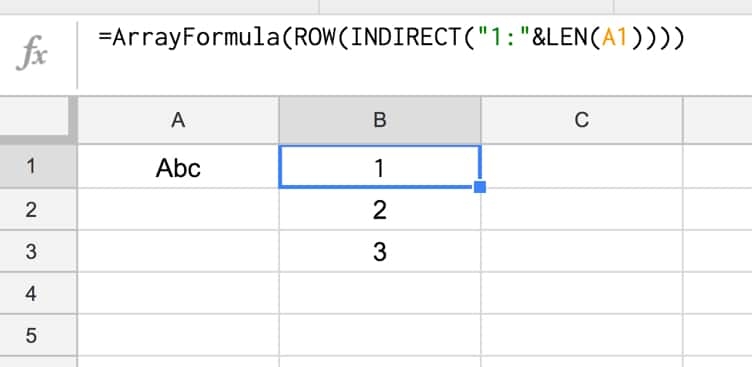Step 3:

Reverse the output, so 3 is in cell B1, 2 in B2 and 1 in B3, by subtracting from the length of the text in A1 and adding 1 to avoid 0:

`=ArrayFormula(LEN(A1)-ROW(INDIRECT("1:"&LEN(A1))) + 1)`

Step 4:

Use the MID formula to now extract the letters at position 3 (“c”), position 2 (“b”) and position 1 (“A”) and display in cells B1:B3:

`=ArrayFormula(MID(A1,LEN(A1)-ROW(INDIRECT("1:"&LEN(A1))) + 1 , 1))`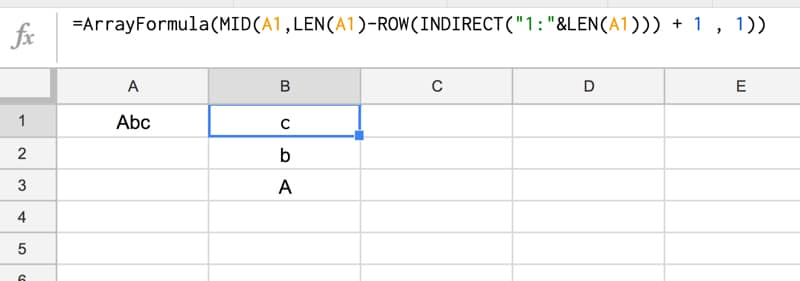Step 5:

Concatenate so that all the individual outputs are combined into a single cell:

`=ArrayFormula(CONCATENATE(MID(A1,LEN(A1)-ROW(INDIRECT("1:"&LEN(A1))) + 1 , 1)))`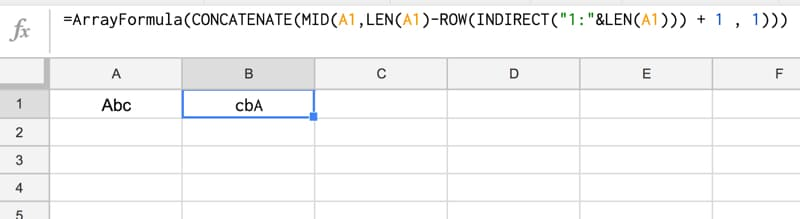Step 6 (optional):

The string is essentially reversed now, so we could stop here.

However, you can use the PROPER function to capitalize the first letters of each word only, for a true reverse effect:

`=ArrayFormula(PROPER(CONCATENATE(MID(A1,LEN(A1)-ROW(INDIRECT("1:"&LEN(A1))) + 1 , 1))))`

Step 7 (optional):

Last step is to add in an IFERROR function to avoid an unsightly error message if the input cell (A1) is blank:

`=ArrayFormula(IFERROR(PROPER(CONCATENATE(MID(A1,LEN(A1)-ROW(INDIRECT("1:"&LEN(A1)))+1,1))),""))`

Final output:

Here’s the formula in action again: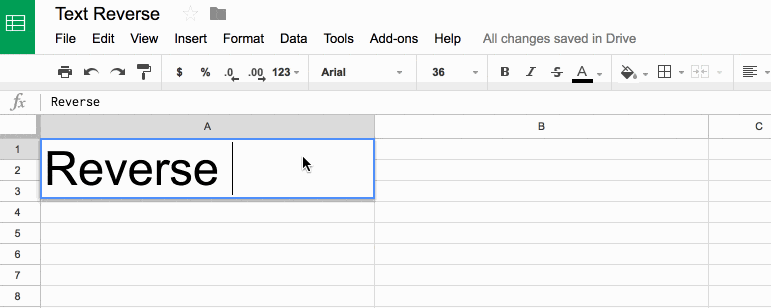## How to reply to Google Forms survey responses quickly with Sheets & Apps Script

You’re happy!

They’re sitting pretty in a Google Sheet but now you’re wondering how you can possibly reply to all those people to say thank you.

Manually composing a new email for each person in turn will take forever. It’s not an efficient use of your time.

You could use an ESP like Mailchimp to send a bulk “Thank You” message, but it won’t be personal. It’ll be a generic, bland email and nobody likes that. It won’t engage your customers and you’ll be missing an opportunity to start a genuine conversation and reply to any feedback from the survey.

Thankfully, there is another way.

Of course there is, otherwise why would I be writing this tutorial! 😉

By adding a reply column to your Google Sheet, next to the Google Forms survey responses, you can efficiently compose a personal response to every single survey respondent.

Then, using Google Apps Script (a Javascript-based language to extend Google Apps, start here if you’re new), you can construct an email programmatically for each person, and send out the responses in bulk directly from your Google Sheet.

## How to Create an Annotated Line Graph in Google Sheets

This post looks at how to make a line graph in Google Sheets, an advanced one with comparison lines and annotations, so the viewer can absorb the maximum amount of insight from a single chart.

For fun, I’ll also show you how to animate this line graph in Google Sheets.

Want your own copy of this line graph?

This chart was originally developed for The Write Life during their 4-day product sale earlier this year. It featured as part of a dashboard that was linked to the E-junkie sales platform and displayed sales data in real-time:

## The data table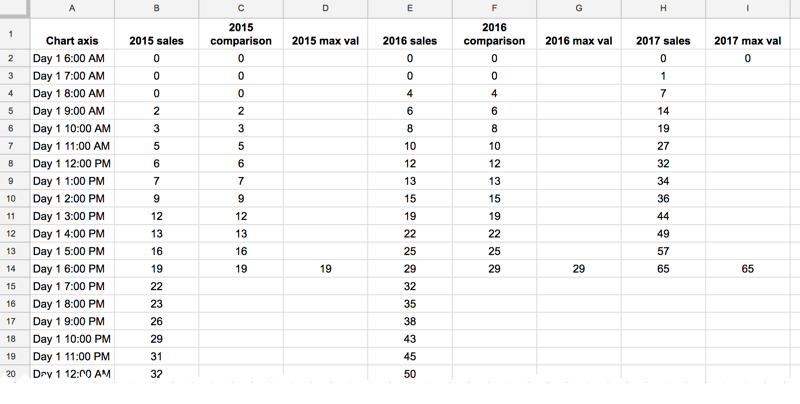The key to this line graph in Google Sheets is setting up the data table correctly, as this allows you to show an original data series (the grey lines in the animated GIF image), progress series lines (the colored lines in the animated GIF) and current data values (the data label on the series lines in the GIF).

In this example, I have date and times as my row headings, as I’m measuring data across a 4-day period, and sales category figures as column headings, as follows: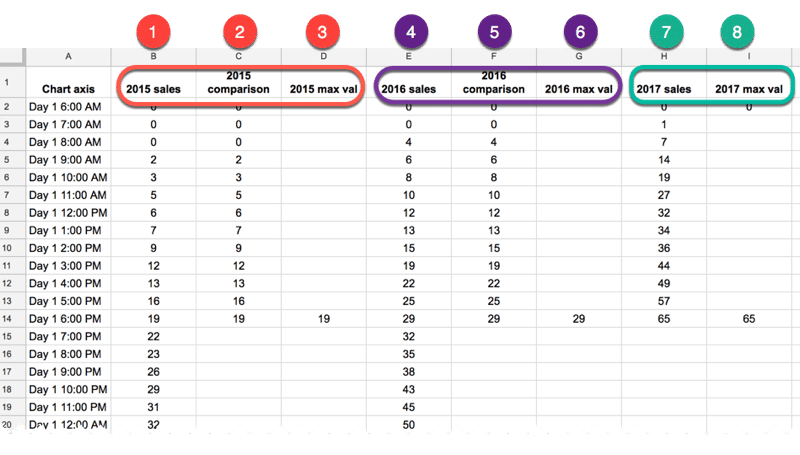### Red columns

The red column, labeled with 1 above, contains historic data from the 2015 sale.

Red column 2 is a copy of the same data but only showing the progress up to a specific point in time.

In red column 3, the following formula will create a copy of the last value in column 2, which is used to add a value label on the chart:

`=IF(AND((C2+C3)=C2,C2<>0),C2,"")`

### Purple columns:

Purple columns 4,5 and 6 are exactly the same but for 2016 data. The formula in this case, in column 6, is:

`=IF(AND((F2+F3)=F2,F2<>0),F2,"")`

### Green columns:

Data in green columns 7 and 8, is our current year data (2017), so in this case there is no column of historic data. The formula in column 8 for this example is:

`=IF(AND((H2+H3)=H2,H2<>0),H2,"")`

## Creating the line graph in Google Sheets

Highlight your whole data table (Ctrl + A if you’re on a PC, or Cmd + A if you’re on a Mac) and select Insert > Chart from the menu.

In the Recommendations tab, you’ll see the line graph we’re after in the top-right of the selection. It shows the different lines and data points, so all that’s left to do is some formatting.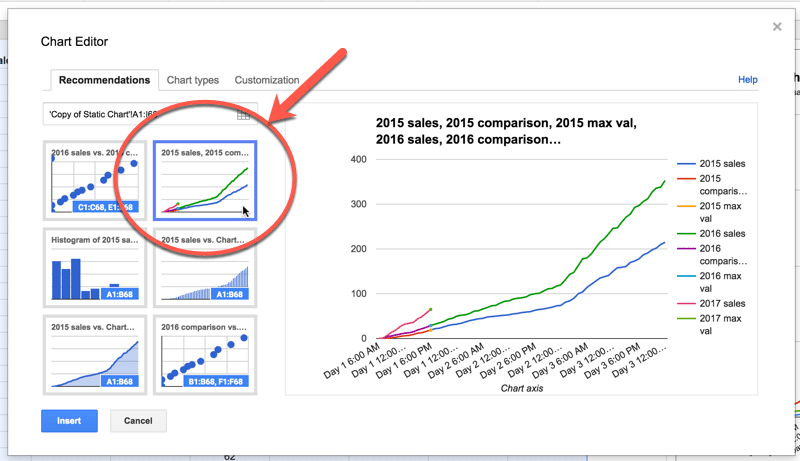Format the series lines as follows:

• For the historic data (columns 1 and 4 in the data table), make light grey and 1px thick
• For the current data (columns 2, 5 and 7 in the data table), choose colors and make 2px thick
• For the “max” values (columns 3, 6 and 8 in the data table), match the current data colors, make the data point 7px and add data label values (see steps 1, 2 and 3 in the image below)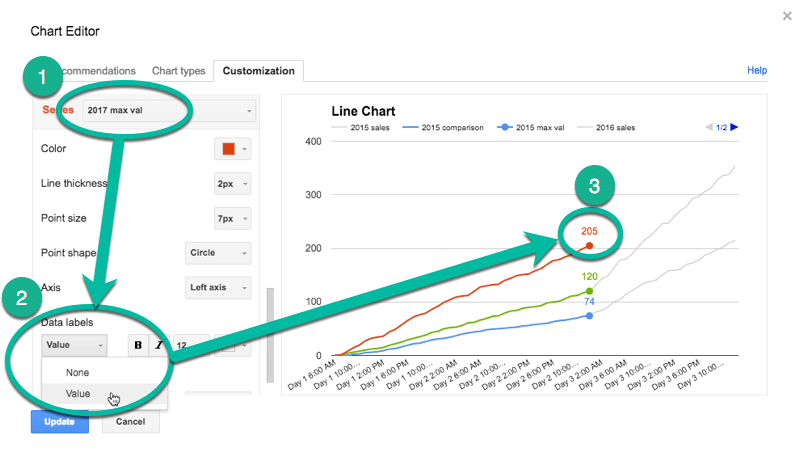This is the same technique I’ve written about in more detail in this post:

How can I annotate data points in Google Sheets charts?

## Animating the chart with Apps Script

How about creating an animated version of this chart?

Oh, go on then.

When this script runs, it collects the historic data, then adds that data back to each new row after a 10 millisecond delay (achieved with the Utilities.sleep method and the SpreadsheetApp.flush method to apply all pending changes).

I don’t make any changes to the graph or create any fancy script to change it, I leave that up to the Google Chart Tool. It just does its best to keep up with the changing data, although as you can see from the GIF at the top of this post, it’s not silky smooth.

By the way, you can create and modify charts with Apps Script (see this waterfall chart example, or this funnel chart example) or with the Google Chart API (see this animated temperature chart). This may well be a better route to explore to get a smoother animation, but I haven’t tried yet…

Here’s the script:

```function startTimedData() {
var sheet = ss.getSheetByName('Animated Chart');
var lastRow = sheet.getLastRow()-12;

var data2015 = sheet.getRange(13,2,lastRow,1).getValues(); // historic data
var data2016 = sheet.getRange(13,5,lastRow,1).getValues(); // historic data

// new data that would be inputted into the sheet manually or from API
var data2017 = [,,,,,,,,,,,,,,,,,,,,,,,,,
,,,,,,,,,,,,,,,,,,,,,,
,,,,,,,,,,,,,,,,,,];

for (var i = 0; i < data2015.length;i++) {
outputData(data2015[i],data2016[i],data2017[i],i);
}

}

function outputData(d1,d2,d3,i) {
var sheet = ss.getSheetByName('Animated Chart');

sheet.getRange(13+i,3).setValue(d1);
sheet.getRange(13+i,6).setValue(d2);
sheet.getRange(13+i,8).setValue(d3);
Utilities.sleep(10);
}

function clearData() {
var sheet = ss.getSheetByName('Animated Chart');
var lastRow = sheet.getLastRow()-12;

sheet.getRange(13,3,lastRow,1).clear();
sheet.getRange(13,6,lastRow,1).clear();
sheet.getRange(13,8,lastRow,1).clear();

}```

On lines 6 and 7, the script grabs the historic data for 2015 and 2016 respectively. For the contemporary 2017 data, I’ve created an array in my script to hold those values, since they don’t exist in my spreadsheet table.

This code is available here on GitHub.

```function onOpen() {